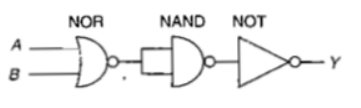A transistor is operated in common-emitter configuration at ${V}_{c}=2volt$ such that a change in the base current from 100 $\mu A$ to 200 $\mu A$ produces a change in the collector current from 5 mA to 10 mA. The current gain is

1. 75                                2. 100

3. 150                              4. 50

Concept Questions :-

Transistor
High Yielding Test Series + Question Bank - NEET 2020

Difficulty Level:

Sodium has body centred packing.Distance between two nearest atom is $3.7\stackrel{0}{A}$. The lattice parameter is

(a) 6.8 $\stackrel{0}{A}$                                     (b) 4.3 $\stackrel{0}{A}$

(c) 3.0 $\stackrel{0}{A}$                                     (d) 8.6 $\stackrel{0}{A}$

Concept Questions :-

Types of semiconductors
High Yielding Test Series + Question Bank - NEET 2020

Difficulty Level:

If the lattice parameter for a crystalline structure is  then the atomic radius in fcc crystal is

(a)                                   (b)

(c)                                 (d)

Concept Questions :-

Types of semiconductors
High Yielding Test Series + Question Bank - NEET 2020

Difficulty Level:

The voltage gain of an amplifier with 9% negative feedback is 10. The voltage gain without feedback will be

(a) 90                            (b) 10

(c) 1.25                         (d) 100

Concept Questions :-

Applications of Transistor
High Yielding Test Series + Question Bank - NEET 2020

Difficulty Level:

A p-n photodiode is made of a material with a band gap of 2.0 eV. The minimum frequency of the radiation that can be absorbed by the material is nearly

1.                               2.

3.                                 4.

Concept Questions :-

Applications of PN junction
High Yielding Test Series + Question Bank - NEET 2020

Difficulty Level:

The circuit is equivalent to1. AND gate                              2. NAND gate

3. NOR gate                              4. OR gate

Concept Questions :-

Logic gates
High Yielding Test Series + Question Bank - NEET 2020

Difficulty Level:

In the forward bias arrangement of a PN-junction diode

(a) The N-end is connected to the positive terminal of the battery

(b) The P-end is connected to the positive terminal of the battery

(c) The direction of current is from N-end to P-end in the diode

(d) The P-end is connected to the negative terminal of battery

Concept Questions :-

PN junction

Difficulty Level:

In a PN-junction diode

1. The current in the reverse biased condition is generally very small

2. The current in the reverse biased condition is small but the forward biased current is independent of the bias voltage

3. The reverse biased current is strongly dependent on the applied bias voltage

4. The forward biased current is very small in comparison to reverse biased current

Concept Questions :-

PN junction
High Yielding Test Series + Question Bank - NEET 2020

Difficulty Level:

The cut-in voltage for silicon diode is approximately

(a) 0.2 V                       (b) 0.6 V
(c) 1.1 V                       (d) 1.4 V

Concept Questions :-

Types of semiconductors

Difficulty Level:

The electrical circuit used to get smooth output from a rectifier circuit is called

(a) Oscillator                          (b) Filter

(c) Amplifier                           (d) Logic gates

Concept Questions :-

Rectifier